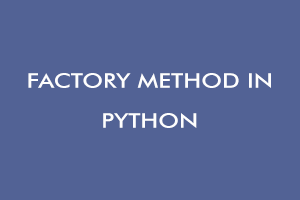Author - StudySection Post Views - 107 views# Factory Method in Python

## Introduction

Design patterns are the tried and tested software design templates that are used to solve problems in software development efficiently. Software developers practice these design patterns to make their code more scalable and maintainable. There are the following three categories of design patterns:

• Creational Patterns
• Structural Patterns
• Behavioral Patterns

Creational Patterns provide a mechanism to create classes and objects in an efficient way to increase the code reusability.
Structural patterns are followed to arrange classes and objects to form a large, flexible structure.
Behavioral Patterns algorithmically explain the communication patterns between objects.

### Factory Method

The factory method is a creational design pattern that provides a common interface for creating objects. It enhances the loose coupling by creating an abstract function (a factory method) that will be used to create different types of objects depending upon the input we give. Instead of writing code to create objects of each and every class we have, now we just call factory methods that will take care of creating objects of the required class.

### Example

In the following example, the function “shape_factory_method” is used to create objects of different shapes. We can extend our code to any type of shape by only adding a class for that shape without tempering the existing code. Here, the factory method ”
```class Circle: def area(radius): output = 3.14 * pow(radius, 2) return("Area of circle =" + str(output)) class Sphere: def area(radius): output = 4* 3.14 * pow(radius, 2) return("Area of sphere =" + str(output)) class Square: def area(side): output = side * side return("Area of square =" + str(output)) class Rectangle: def area(length,width): output = length * width return("Area of rectangle =" + str(output)) def shape_factory_method(shape = "circle"): shape = str(shape).lower() shape_obj = { "circle":Circle, "sphere":Sphere, "square":Square, "rectangle":Rectangle } return shape_obj[shape] if __name__ == "__main__": shape_object = shape_factory_method("sphere") result = shape_object.area(6) print(result) shape_object = shape_factory_method("square") result = shape_object.area(100) print(result)```